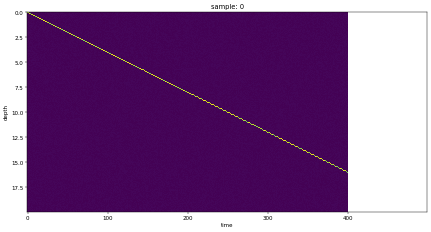# How to use HoloMap to animate x-axis to view a window into a larger image being panned?

Hi all,

I am trying to generate an animation (.webm video rendering using matplotlib backend) using a HoloMap that displays a subset of a much larger holoviews.Image() and pans across the entire image over time. This is meant to look like a normal realtime sonar display.

The issue I am having is that the HoloMap seems to define the x-axis as the union of the individual image coordinates instead of just the current image coordinates.

Ideally I want the first frame of the video be showing the x-axis as values 0 - 400 (Sorry was unable to upload extra images to show the issue)

and the last frame of the video be showing the x-axis as values 100 - 500

But instead in the video the x-axis covers the entire range from 0 - 500 and the image has white “empty” areas. I.e. It pans the data and not the axis. Is there some way to get HoloMap to do what I am after?I tried to attach the .webm video but was unable to so uploaded a still of the first frame generated instead.

Thanks,
Brendon.

Below is the code to reproduce the issue:

``````WIDTH = 400
HEIGHT = 200
MAX_DEPTH = 20

DATA_POINTS = WIDTH + 100
SONAR_BINS = HEIGHT
VIDEO_FRAMES = DATA_POINTS - WIDTH + 1

import holoviews
import xarray
import numpy.random
import collections

holoviews.extension('matplotlib')

# Real data comes from a sonar log file, this is just made up to make the
# example standalone with data and a solid linear depth to make it easier
# to see the image panning
time_arr = range (0, DATA_POINTS)
sonar_data_arr = numpy.random.randint(0, 5, (DATA_POINTS, SONAR_BINS))
for i in range(0, len(time_arr)):
bottom_depth_bin = int(i / float(len(time_arr)) * SONAR_BINS)
sonar_data_arr[i][bottom_depth_bin] = 200 + numpy.random.randint(0, 55)
#if bottom_depth_bin < SONAR_BINS - 1:
#	sonar_data_arr[i][bottom_depth_bin+1] = 200 + numpy.random.randint(0, 55)

channel = xarray.Dataset({
'amplitudes': (['time', 'depth'], sonar_data_arr, {'units': 'amplitude'}),
},
coords={
'time': (['time'], time_arr),
'depth': (['depth'], [i * (float(MAX_DEPTH) / SONAR_BINS) for i in range(0,SONAR_BINS)]),
})
ds = holoviews.Dataset(channel)

hmap_dict = collections.OrderedDict()
for x_start in range(0,VIDEO_FRAMES):
print (str(x_start) + ' of ' + str(VIDEO_FRAMES))
x_range = (x_start, x_start + WIDTH)
img = ds.select(time=x_range).to(holoviews.Image, kdims=["time", "depth"])
img = img.opts(cmap='viridis', logz=False, invert_yaxis=True)

# Matplotlib plotting sizes are VERY different to bokeh: http://holoviews.org/user_guide/Plotting_with_Matplotlib.html
img = img.opts(aspect=float(WIDTH) / float(HEIGHT), fig_inches=10, fig_bounds=(0, 0, 1, 1))
holoviews.output(fig='png', dpi=int(WIDTH/10))

hmap_dict[x_start] = img
hmap = holoviews.HoloMap(hmap_dict, kdims='sample')

# Create a video using the matplotlib .webm rendering of a HoloMap
print ('Creating .webm video')
holoviews.output(fig='png', dpi=int(WIDTH))
holoviews.save(hmap, 'img_first.png', backend='matplotlib')
holoviews.save(hmap[VIDEO_FRAMES-1], 'img_last.png', backend='matplotlib')

holoviews.output(fig='png', dpi=int(WIDTH/10))
holoviews.save(hmap, 'img.webm', backend='matplotlib', fps=20)``````